### Areas Related To A Circle Class 10th Mathematics Gujarat Board Solution

##### Question 1.The area of a circular park is 616 m2. There is a 3.5 m wide track around the park running parallel to the boundary. Calculate the cost of fencing on the outer circle at the rate of Rs. 5 per meter.Answer:Area of the circular park = 616 m2width of the track = 3.5 m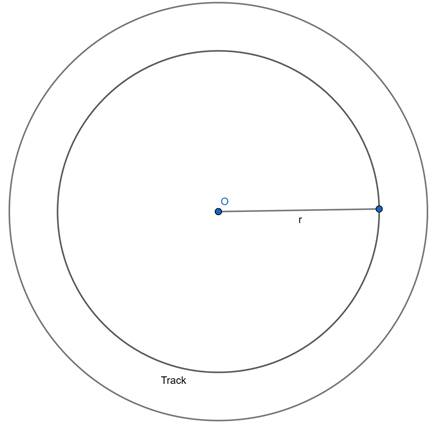Area of the circular park = 616 m2π × r2 = 616r2 = 196r = √196r = 14 m∴ the radius of the circular park (r1) = 14 mthe radius of the park with track (r2) = 14 + 3.5= 17.5 mCircumference of the track = 2π (r2)= 2×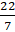(17.5)= 110 mCost of fencing per meter = Rs. 5Cost of fencing 110 m = 110 × 5= 550Question 2.A man is cycling in such a way that the wheels of the cycle are making 140 revolutions per minute. If the diameter of the wheel is 60 cm, then how much distance will he cover in 2 hours?Answer:Speed of the cycle = 140RPMDiameter of the wheel = 60 cmNo. of revolution in 2 hours = 120 × 140= 16,800Distance covered in 2 hours = Revolutions × Diameter= 16,800 × 60= 1008000 cm= 10080 m= 10.8 kmQuestion 3.If a chord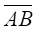of (O, 20) subtends right angle at O, find the area of the minor segment.Answer:Given radius = 20cmif we connect the chord to the center point that forms a segment.the centre measure angle (θ) =90owe can observe a right-angled triangle OAB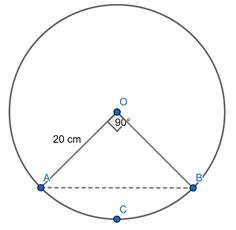Area of segment =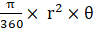=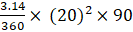= 314 cm2Area of the triangle =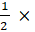OA× OB=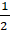× (20) × (20)= 200 cm2Area of minor segment = Area of segment - Area of the triangle= 314 – 200= 114 cm2Question 4.There are two arcs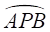of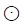(O, OA) and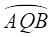of(M, MA) as shown in figure 13.24. Find the area enclosed by two arcs.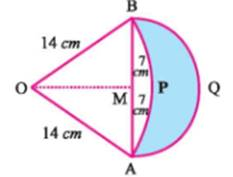Figure 13.24Answer:from the figure we can notice all these1. OAB is an equilateral triangle(∵ All the sides are equal)2. OAPB is a minor segment with Radius 14 cm, the angle (θ) between them is 60o3. ABQ is a semi-circle with centre as M radius 7 cmArea of the segment ==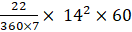= 102.62 CM2Area of the triangle =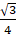× OA2=× 142= 84.870 CM2Area of the semi-circle =Area of the segment - Area of the triangle= 102.62 - 84.870= 17.75 cm2Area of the semi-circle =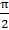× r2=× 72= 76.96 cm2area enclosed by two arcs =Area of the semi-circle - Area of the semi-circle= 76.96 – 17.75= 59.20 cm2 (approximately)Question 5.The length of a side of a square garden ABCD is 70 m. A minor segment of(O, OA) is drawn on each of two opposite sides for developing lawn, as shown in figure 13.25. Find the area of the lawn.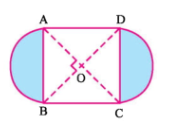figure 13.25Answer:side of a square garden ABCD (a) = 70 mFrom the fig. in the below we can see that1. OAXD is a segment2. OAD is an right-angled triangle.3. AXD is a minor segmentOA, OD are the radii of the segmentfrom the triangle OADAD2 = OA2 + OD2702 = r2 + r22r2 = 4900r2 = 2450r = √2450Area of minor segment = Area of segment - Area of triangle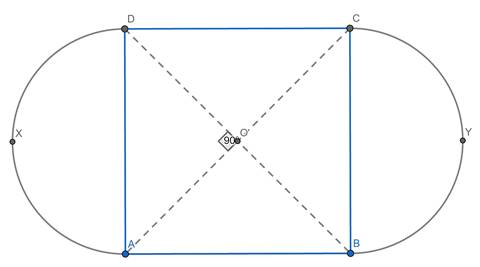=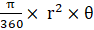-× OA2=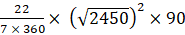-×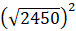= 1924.22 – 1225=699.22 cm2= 700 cm2since AXD, BYC are same∴ Area of lawn = 2 × area of minor segment= 2 × 700= 1400 cm2Question 6.ABCD is a square of side 20 cm. Find the area of blue coloured region formed by the semi-circles drawn on each side as shown in figure 13.26. ( = 3.14)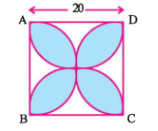figure 13.26.Answer:side of a square (a) = 20 cmwe can see the semi-circle in the square having 10 cm as radius (r)area of the colored region =area of 4 semi-circles - area of the square= 4 × (× r2) - a2= 2 (π × 102) - 202= 228 cm2Question 7.On a circular table top of radius 30 cm a design is formed leaving an equilateral triangle inscribed in a circle. Find the area of the design. (π = 3.14)Answer:radius of the circle = 30 cmArea of the circle = π r2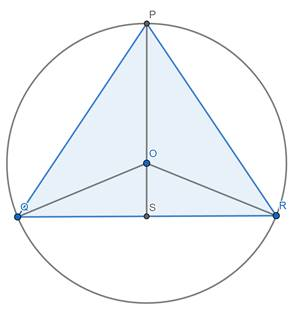= 3.14 × 302= 2826 cm2PQR is an equilateral triangle∠ QPR = 60O∠ QOR = 2 × ∠ QPR = 120oIn OQR triangle,let OS is perpendicular to QRIn triangle OQR as OQ = OR∠ QOS =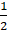∠ QPR = 60OIn triangle OSQ ∠S = 90osin 60o =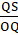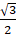=QS = 15 √3 cmNow,QR = 2 QS = 2 (15 √3) = 30√3 cmArea of equilateral triangle =× QR2=× (30√3)2= 1167.75 CM2Area of design = Area of circle - area of triangle PQR= 2826 – 1167.75= 1658.25 CM2Question 8.Figure 13.27 shows a kite formed by a square PQRS and an isosceles right triangle ARB whose congruent sides are 5 cm long.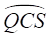is an arc of a(R, 42). Find the area of the blue coloured region.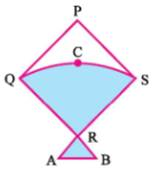Figure 13.27Answer:Side of square (r) = 42 cmIn triangle RAB,RA = RB = 5 cmIn minor sector RQCS angle is 90oArea of the shaded region = Area of minor sector RQCS + Area of RAB=+× RA × RB=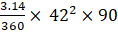+× 5 × 5= 1384.74 + 12.5= 1372.24 cm2Question 9.In figure 13.28, ABCD is a square with sides having length 8 cm. Find the area of the blue coloured region. (π = 3.14)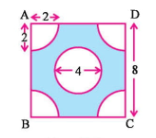Figure 13.28Answer:side of square (a) = 8 cmfrom the fig. we can see that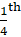of circle is placed at four parts of the square. if we join them a circle is formed with radius (r1) 2 cmAnother circle is placed at centre of the square with radius (r2) 2 cmArea of colored region = Area of square - Area of circles at 4 corners + Area of circle at centre= a2 – (4 × (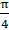× r12) + (π r22))= a2 – (π r12 + π r22)= 82 – (3.14 (22 + 22))= 38.88 cm2Question 10.A circle is inscribed in APQR where m∠Q = 90, PQ = 8 cm and QR = 6 cm. Find the area of the blue coloured region shown in figure 13.29. (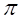= 3.14)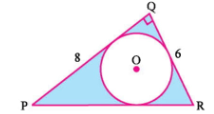Figure 13.29Answer:from the given figurePQR is a right-angle trianglePQ = 8 cmQR = 6 cmPR2 = PQ2 + QR2PR2 = 82 + 62PR = √100 = 10 cmRadius of the incircle (r) =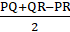=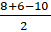== 2 CMarea of coloured region = Area of triangle - area of circle=× PQ × QR - π r2=× 8 × 6 - π 22= 24 – 12.566= 11.433 cm2Question 11.Select a proper option (a), (b), (c) or (d) from given options and write in the box given on the right so that the statement becomes correct:If an arc of a circle subtends an angle of measure 0 at the centre, then the area of the minor sector is…..A.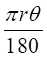B.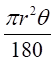C.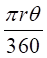D.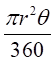Answer:we know that area of minor sector =So, option(d) is the correct answer.Question 12.Select a proper option (a), (b), (c) or (d) from given options and write in the box given on the right so that the statement becomes correct:The area of a sector is given by the formula ….(r is the radius and 1 is the length of an arc.)A.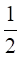rlB.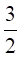r2/ lC.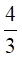rlD.rlAnswer:we know that when arc length (l) and radius (r) of sector is givenArea of the sector is =rlSo, option(a) is the correct answer.Question 13.Select a proper option (a), (b), (c) or (d) from given options and write in the box given on the right so that the statement becomes correct: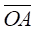and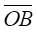are the two mutually perpendicular radii of a circle having radius 9 cm. The area of the minor sector corresponding to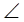AOB is cm2(= 3.14)A. 63.575B. 63.585C. 63.595D. 63.60Answer:Given radius (r)= 9 cm∵ They are perpendicular to each other θ = 90oarea of the minor sector ==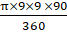=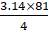= 63.585 cm2So, option(b) is the correct answer.Question 14.Select a proper option (a), (b), (c) or (d) from given options and write in the box given on the right so that the statement becomes correct:A sector subtends an angle of measure 120 at the centre of a circle having radius of 21 cm. The area of the sector is ……….. cm2.A. 462B. 460C. 465D. 470Answer:Given radius (r)= 21 cmangle(θ) = 120oarea of the minor sector ==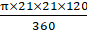=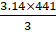= 461.58 cm2= 462 cm2So, option(a) is the correct answer.Question 15.Select a proper option (a), (b), (c) or (d) from given options and write in the box given on the right so that the statement becomes correct:If the area and the circumference of a circle are numerically equal, then r = …………A. πB.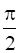C. 1D. 2Answer:Area of the circle = πr2circumference of the circle = 2πrgiven, Area = Circumference2πr = πr22 = rSo, option(d) is the correct answer.Question 16.Select a proper option (a), (b), (c) or (d) from given options and write in the box given on the right so that the statement becomes correct:The length of an arc subtending an angle of measure 60 at the centre of a circle whose area is 616 is ……….A.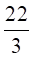B. 66C.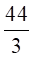D. 33Answer:Area of circle = 616cm2πr2 = 616r2 = 196r = 14 cmGiven θ = 60oarea of the minor sector ==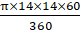=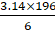=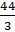cm2So, option(c) is the correct answer.Question 17.Select a proper option (a), (b), (c) or (d) from given options and write in the box given on the right so that the statement becomes correct:The area of a minor sector of (O, 15) is 150. The length of the corresponding arc is (π = 3.14)A. 30B. 20C. 90D. 15Answer:Radius of the minor sector= 15 cmArea of minor sector = 150Area of a minor sector =r l150 =× 15 × ll = 20 cmSo, option(b) is the correct answer.Question 18.Select a proper option (a), (b), (c) or (d) from given options and write in the box given on the right so that the statement becomes correct:If the radius of a circle is increased by 10 %, then corresponding increase in the area of the circle is ………. (π = 3.14)A. 19 %B. 10 %C. 21 %D. 20 %Answer:r be the radius of the circleArea of the circle = πr210% increase in radius = 1.1rArea of circle with new radius = π(1.1r)2= 1.21πr2increase in area = 1.21πr2 - πr2= 0.21 πr2∴ 21% increase in the area of circleSo, option(c) is the correct answer.Question 19.Select a proper option (a), (b), (c) or (d) from given options and write in the box given on the right so that the statement becomes correct:If the ratio of the area of two circles is 1: 4, then the ratio of their circumference …..A. 1: 4B. 1: 2C. 4: 1D. 2: 1Answer:let r1, r2 are the radii of two circlesgiven,πr12: πr22 = 1 : 4r12: r22 = 1 : 4r1 : r2 =1 : 2r2 = 2 r1circumference of 1st circle = 2πr1circumference of 2nd circle = 2πr2 = 2π(2r1) = 4πr1ratio of circumference = 2πr1 : 4πr1 = 1 : 2So, option(b) is the correct answer.Question 20.Select a proper option (a), (b), (c) or (d) from given options and write in the box given on the right so that the statement becomes correct:The area of the largest triangle inscribed in a semi-circle of radius 8 is ……A. 8B. 16C. 64D. 256Answer:Given radius of semi-circle = 8 cmFor a largest triangle in semi-circlebase will be its diameter of semi-circleheight will be radius of semi-circleso, its base = 2 × radius = 2 × 8 = 16 cmheight = 8 cmArea of the triangle =× base × height=× 16 × 8= 64 cm2So, option(c) is the correct answer.Question 21.Select a proper option (a), (b), (c) or (d) from given options and write in the box given on the right so that the statement becomes correct:If the circumference of a circle is 44 then the length of a side of a square inscribed in the circle is ….A.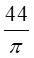B.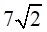C.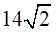D.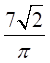Answer:Given circumference = 44 cm2πr = 44πr = 22r = 7 cmGiven that square inscribed in a circle.so, diagonal of square will be equal to diameter of circle.diagonal = 2 × radius = 2 × 7 = 14 cmdiagonal = √2 × side of square14 = √2 × sideside = 7√2 cmQuestion 22.Select a proper option (a), (b), (c) or (d) from given options and write in the box given on the right so that the statement becomes correct:The length of minute hand of a clock is 14 cm. If the minute hand moves from 2 to 11 on the circular dial, then area covered by it is cm2.A. 154B. 308C. 462D. 616Answer:The angle moved by minute hand = 90olength of minute hand (r) = 14 cmArea of covered by minute hand =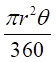=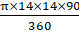=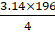= 153.93 cm2= 154 cm2So, option(a) is the correct answer.Question 23.Select a proper option (a), (b), (c) or (d) from given options and write in the box given on the right so that the statement becomes correct:The length of minute hand of a clock is 15 cm. If the minute hand moves for 20 minutes on a circular dial of a clock, the area covered by it is …….. cm2. (= 3.14)A. 235.5B. 471C. 141.3D. 706.5Answer:The angle moved by minute hand = 120olength of minute hand (r) = 15 cmArea of covered by minute hand ==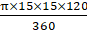=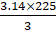= 235.619 cm2= 235.5 cm2So, option(a) is the correct answer.

PDF FILE TO YOUR EMAIL IMMEDIATELY PURCHASE NOTES & PAPER SOLUTION. @ Rs. 50/- each (GST extra)

HINDI ENTIRE PAPER SOLUTION

MARATHI PAPER SOLUTION

SSC MATHS I PAPER SOLUTION

SSC MATHS II PAPER SOLUTION

SSC SCIENCE I PAPER SOLUTION

SSC SCIENCE II PAPER SOLUTION

SSC ENGLISH PAPER SOLUTION

SSC & HSC ENGLISH WRITING SKILL

HSC ACCOUNTS NOTES

HSC OCM NOTES

HSC ECONOMICS NOTES

HSC SECRETARIAL PRACTICE NOTES

# 2019 Board Paper Solution

HSC ENGLISH SET A 2019 21st February, 2019

HSC ENGLISH SET B 2019 21st February, 2019

HSC ENGLISH SET C 2019 21st February, 2019

HSC ENGLISH SET D 2019 21st February, 2019

SECRETARIAL PRACTICE (S.P) 2019 25th February, 2019

HSC XII PHYSICS 2019 25th February, 2019

CHEMISTRY XII HSC SOLUTION 27th, February, 2019

OCM PAPER SOLUTION 2019 27th, February, 2019

HSC MATHS PAPER SOLUTION COMMERCE, 2nd March, 2019

HSC MATHS PAPER SOLUTION SCIENCE 2nd, March, 2019

SSC ENGLISH STD 10 5TH MARCH, 2019.

HSC XII ACCOUNTS 2019 6th March, 2019

HSC XII BIOLOGY 2019 6TH March, 2019

HSC XII ECONOMICS 9Th March 2019

SSC Maths I March 2019 Solution 10th Standard11th, March, 2019

SSC MATHS II MARCH 2019 SOLUTION 10TH STD.13th March, 2019

SSC SCIENCE I MARCH 2019 SOLUTION 10TH STD. 15th March, 2019.

SSC SCIENCE II MARCH 2019 SOLUTION 10TH STD. 18th March, 2019.

SSC SOCIAL SCIENCE I MARCH 2019 SOLUTION20th March, 2019

SSC SOCIAL SCIENCE II MARCH 2019 SOLUTION, 22nd March, 2019

XII CBSE - BOARD - MARCH - 2019 ENGLISH - QP + SOLUTIONS, 2nd March, 2019

# HSCMaharashtraBoardPapers2020

(Std 12th English Medium)

HSC ECONOMICS MARCH 2020

HSC OCM MARCH 2020

HSC ACCOUNTS MARCH 2020

HSC S.P. MARCH 2020

HSC ENGLISH MARCH 2020

HSC HINDI MARCH 2020

HSC MARATHI MARCH 2020

HSC MATHS MARCH 2020

# SSCMaharashtraBoardPapers2020

(Std 10th English Medium)

English MARCH 2020

HindI MARCH 2020

Hindi (Composite) MARCH 2020

Marathi MARCH 2020

Mathematics (Paper 1) MARCH 2020

Mathematics (Paper 2) MARCH 2020

Sanskrit MARCH 2020

Sanskrit (Composite) MARCH 2020

Science (Paper 1) MARCH 2020

Science (Paper 2)

Geography Model Set 1 2020-2021

MUST REMEMBER THINGS on the day of Exam

Are you prepared? for English Grammar in Board Exam.

Paper Presentation In Board Exam

How to Score Good Marks in SSC Board Exams

Tips To Score More Than 90% Marks In 12th Board Exam

How to write English exams?

How to prepare for board exam when less time is left

How to memorise what you learn for board exam

No. 1 Simple Hack, you can try out, in preparing for Board Exam

How to Study for CBSE Class 10 Board Exams Subject Wise Tips?

JEE Main 2020 Registration Process – Exam Pattern & Important Dates

NEET UG 2020 Registration Process Exam Pattern & Important Dates

How can One Prepare for two Competitive Exams at the same time?

8 Proven Tips to Handle Anxiety before Exams!